# Nodal Analyses Reference¶

## Introduction¶

In the following discussion we assume that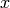is the vector of nodal variables. We denote matrices and frequency-domain quantities with uppercase letters.

This section documents the formulation used for nodal-based analyses. For all analyses there are four types of devices to consider:

Linear VCCS/QS

Contribute the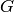and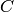matrices, respectively. Elements provide the following attributes to build these matrices: linearVCCS and linearVCQS.

Nonlinear VCCS/QS

Contributeand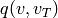vector functions. Here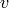is a vector with controlling port voltages and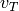is a vector with time-delayed control voltages. For the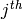nonlinear device, vectorand its Jacobian are returned by eval_and_deriv(). The Jacobian is returned in a single matrix with the following format: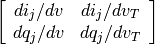In nodal analyses, controlling port voltages () are obtained as differences between elements of the nodal variables vector (). In compact form this can be denoted as:whereis an incidence matrix. All nonzero entries in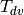are either equal to 1 or -1 and there are at most two nonzero entries per row. This matrix is implicitly stored in the simulator with the control port definitions in each element. Samples of time-delayed control voltages can be calculated in a similar way using aincident matrix but the effect of time delays depends on the analysis type.

Vector functionsandare mapped into the nodal equations using incidence matricesand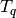, which have the same properties as the transpose of.

Frequency-defined devices

Contribute the complex, frequency-dependent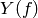matrix. The corresponding impulse-response matrix is denoted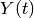. The following methods are used to build this matrix: get_Y_matrix() for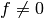and get_G_matrix() for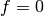. More details will be provided when time-domain support for this kind of device is implemented.

Sources

Contribute a source vector. There are 3 types of source: DC (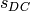), time-domain (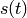) and frequency-domain (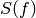). The following element methods are used: get_DCsource(), get_TDsource(), get_FDsource() and get_AC().

## OP/DC Equations¶

For DC analysis, time-delayed control voltages are calculated as regular control voltages;andare ignored and only the DC component of sources () and the DC conductance matrix of frequency-defined elements (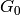) are considered.

The analysis solves the following nonlinear equation iteratively using Newton’s method: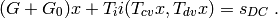Let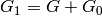. The iteration is defined by linearizingas follows: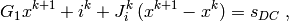where the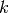superscript indicates the iteration number and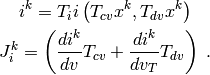Thevector is assumed to be known for each iteration and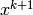is the unknown to solve for. The initial guess (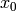) is set to the values suggested by the nonlinear devices, if any, or otherwise to zero. The previous equation can be re-arranged as as the following system of linear equations: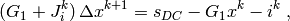with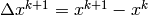. This equation can be seen as the nodal equation of a linearized circuit obtained by substituting all devices by transconductances in parallel with current sources that are dependent of the current approximation for the nodal voltages ().

The condition to stop Newton iterations is: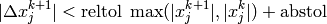for each nodal variable (). In addition, if errfunc is set to True, nodal equations are also checked: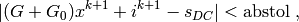and iterations stop when both conditions are satisfied. Normally the first condition is enough to obtain an acceptable solution and that is why by default the second check is not enabled to save the CPU time required to re-evaluate the nodal equations.

## AC Formulation¶

Here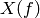is the nodal vector in frequency domain. A DC operating point analysis is performed first to obtain the incremental conductances and capacitances given byand, respectively. The analysis solves forfor all requested frequencies using the following equation: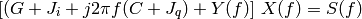The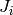and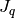Jacobian matrices are calculated as follows:where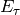is a diagonal matrix that includes the effect of time delays in each control port: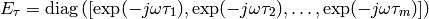## Transient Analysis Equations¶

Transient analysis solves the following nonlinear algebraic-integral-differential equation given an initial condition,: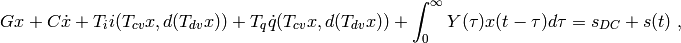where dotted quantities indicate derivative with respect to time and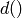is a vector function that applies a (possibly different) time delay to each control voltage. The delay function () is implemented by storing all time-delayed control port voltages and using an interpolation function to find the voltage at the desired time in the past.

The initial condition (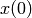) is usually obtained by calculating the operating point of the circuit using the OP analysis.

An integration method such as Backward Euler (BE) or trapezoidal rule is applied to transform the differential equation into a difference equation by discretizing time and approximating derivatives with respect to time. Here we assume the time step (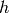) is constant. For example, using the BE rule: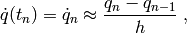here, the subscript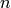denotes the time sample number. For implicit methods in general,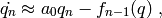with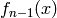being a function that depends on the previous samples of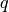: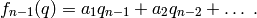The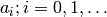coefficients depend on the time step size and the integration method. Substituting dotted variables and discretizing the convolution operation the resulting circuit equation is the following: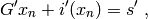with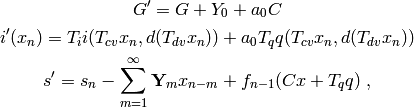where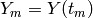and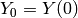. Note that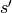is a known vector at the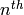time step. This is the equation of a DC circuit with a conductance matrix equal to, a set of nonlinear currents given by the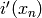function and a source vector given by. The unknown (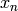) is iteratively solved using Newton’s Method (similarly as in OP/DC analysis). Iterations are defined by linearizing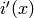as follows: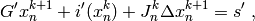where thesubscript denotes the Newton iteration number,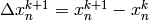and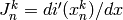. This equation is re-arranged as follows: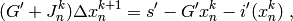as the right-hand side of this equation is known at the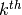iteration,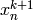can be found by solving a linear system of equations.

The criterion to stop iterations is the same as in the DC analysis.